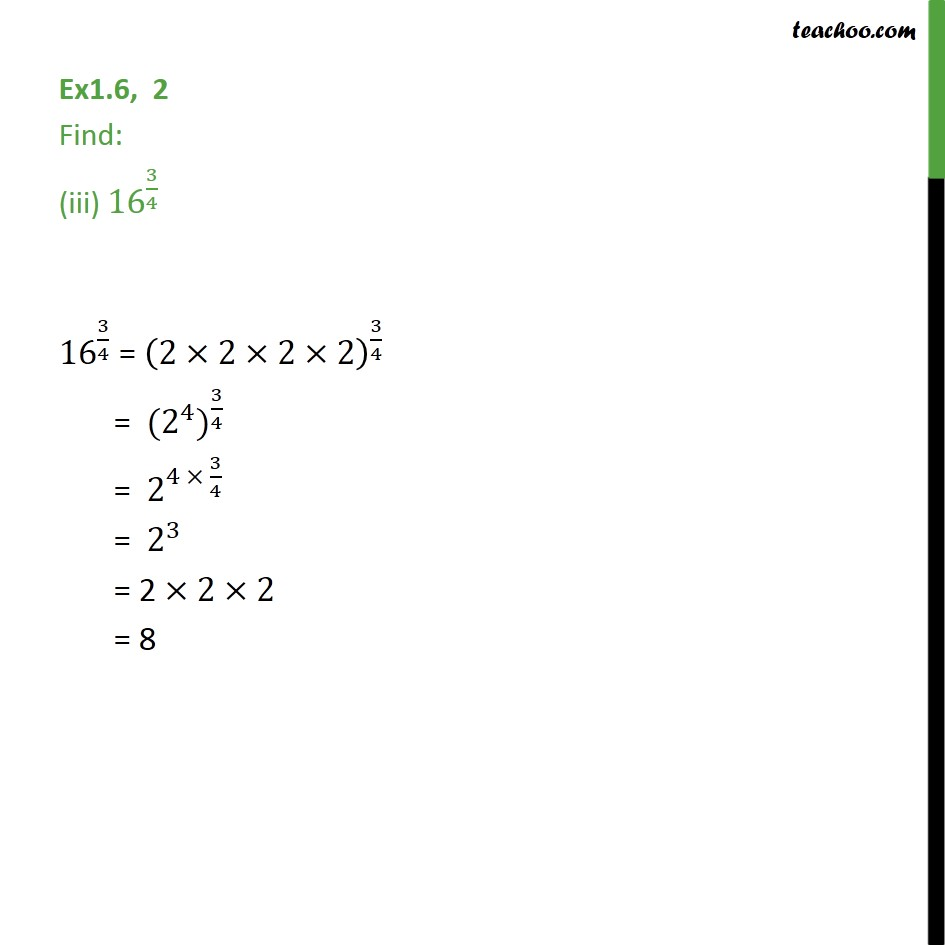1. Chapter 1 Class 9 Number Systems (Term 1)
2. Serial order wise
3. Ex 1.6

Transcript

Ex1.6, 2 Find: (iii) 〖16〗^(3/4) 〖16〗^(3/4) = (2×2×2×2)^(3/4) = 〖 (2^4)〗^(3/4) = 〖 2〗^(4 × 3/4) = 〖 2〗^3 = 2 ×2×2 = 8